# Difference between revisions of "849: Complex Conjugate"

 Complex Conjugate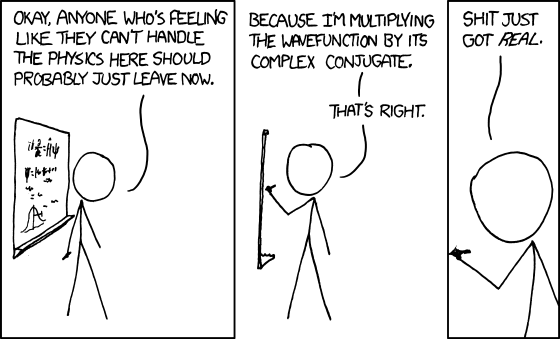Title text: Fun fact: if you say this every time a professor does something to a complex-number equation that drops the imaginary part, they'll eventually move the class to another room and tell everyone else except you.

## ExplanationThis explanation may be incomplete or incorrect: The Schrodinger wave equation needs explaining.If you can address this issue, please edit the page! Thanks.

This comic is a joke on the phrase "Shit just got real", which means "something has suddenly increased in difficulty and become genuinely challenging or dangerous".

Cueball is standing in front of a board delivering a lesson, and is about to multiply a wavefunction by its complex conjugate. A wave function is a mathematical description of a quantum system which uses complex values - numbers that have both a real part and an imaginary part.

Complex numbers can be written in the form$a + bi$, where$a$ is the real part and$bi$ is the imaginary part.$i$ is the imaginary unit, defined to be the square root of$-1$.

The complex conjugate of a complex number simply reverses the sign on the imaginary part - so for the number above, the complex conjugate is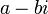$a - bi$.

Multiplying the complex number by its own complex conjugate therefore yields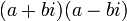$(a + bi)(a - bi)$. If you multiply out the brackets, you get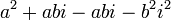$a^2 + abi - abi - b^2i^2$. The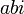$abi$ cancel each other out, and because$i = \sqrt{-1}$, this means that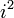$i^2$ is simply$-1$.

Thus, the result is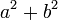$a^2 + b^2$; the imaginary part has completely disappeared, leaving nothing but a real number, so "shit has got real" as Cueball promised.

The title text notes that you can make this joke in class every time a calculation is performed that drops the imaginary part from a complex number, but warns that it would be so annoying that the professor will eventually find a way to have the class without you in it. As complex numbers are often used for calculations, while more seldom finding real life applications, it is not uncommon that towards the solution of a problem, their imaginary parts are dropped again.

The wave function shown on Cueball's board is the time-dependent Schrödinger equation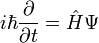$i\hbar\dfrac{\partial}{\partial t} = \hat{H}\Psi$

## Transcript

[Cueball is standing at a whiteboard covered in equations, one of which is the time dependent Schrodinger equation.]
Cueball: Okay, anyone who's feeling like they can't handle the physics here should probably just leave now.
Cueball: Because I'm multiplying the wavefunction by its complex conjugate.
Cueball: That's right.
[Dramatic zoom. It appears Cueball is writing on the side of the panel.]
Cueball: Shit just got real.add a comment! ⋅add a topic (use sparingly)! ⋅refresh comments!

# Discussion

Actually multiplying complex number (x + iy) by its complex conjugate (x - iy) does not "remove" imaginary part, but calculate square of absolute value of complex number, (x^2 + y^2). BTW. in quantum physics the wavefunction is complex valued, and its absolute value is probability density (a real valued function). --JakubNarebski (talk) 00:57, 18 December 2012 (UTC)

I got hit in the face with my complex conjugate and lost an eye. 108.162.238.114 (talk) (please sign your comments with ~~~~)

I procreated with my complex conjugate and lost myself. 108.162.216.114 19:44, 12 August 2014 (UTC)

(a+bi)*(a-bi)= a^2-b^2 not a^2+b^2 108.162.218.142 15:48, 24 June 2015 (UTC)

(a+b)*(a-b) = a^2 - b^2 . However (a+bi)*(a-bi) = a^2 + b^2 since i^2 = -1. 108.162.219.65 (talk) (please sign your comments with ~~~~)

I think that the moving to a title text is a joke about them kicking you out of the room 173.245.50.65 20:54, 1 May 2016 (UTC)

Is it relevant that when he performs the complex conjugate multiplication, we go from a 2d image of the board to a 1d image of the board? My thinking is, that complex numbers are often used to describe a set of coordinates in 2d space. 162.158.158.233 (talk) 06:58, 14 January 2020 (please sign your comments with ~~~~)

It might be a nice coincidence, or happened on purpose. However in my understanding and the usecases I've seen for example in electrical engineering, it was the other way round. Complex Numbers were used to describe something , e.g. phase shift, and then it could be visualized as coordinates where it was visible as a sinus-curve. (I Might be slightly wrong about the details here. It's been some time...) --Lupo (talk) 07:18, 14 January 2020 (UTC)

What's with that red text? Is that MML? ⟨Winter is coming⟩ Marethyu (talk) 21:27, 5 May 2022 (UTC)